# How Many Triangles 1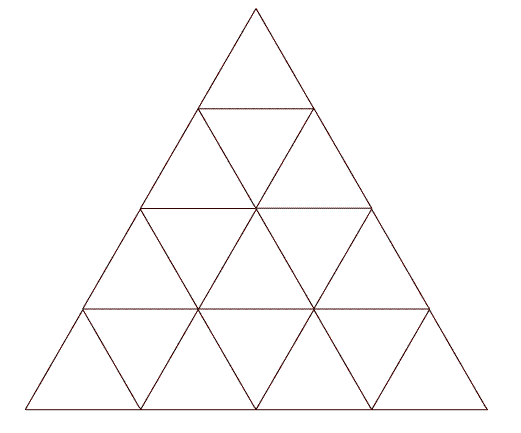How many triangles can you count in this diagram?

Click the yellow button to find out.

## A Mathematics Lesson Starter Of The Day

Topics: Starter | Combinations | Problem Solving | Shape

• Judy, Chatsmore CHS
•
• This triangle starter is excellent. I have used it with all of my ks3 and ks4 classes and they are all totally focused when counting the triangles.
• Jo Melville, Aberdeen
•
• All my S1 - S4 classes enjoyed this activity at different levels. S3 have managed to write a formula for the number of triangles in an n-row triangle. Excellent!
• Guy Broster, Cumbria, UK
•
• A great all-purpose starter for any lesson. Got my little ones on task and ready for their lesson.
• Mr R. Ditta, Stokesley School, North Yorkshire
•
• It is a great starter that could be improved if the demonstration counted along with the shading of the different triangles.
• Year 8 and Miss D, Wade Deacon High school, Widnes
•
• Good starter, gets the mind working. Challenging for a class of year 8's, but fun.
• Mrs Myers' Year 8 gang!, Woldgate 'the best' College
•
• My Year 8 class got 27!!! It did take us a while though!
• Mr Sambhi, Solihull
•
• Excellent starter. I have used with every class I teach.
• Mr Cansdale, 7H3
•
• We really enjoyed this activity, we want more of these please!
• Miss Pankhurst, Norfolk
•
• Excellent starter! I have used it with nearly all of my groups and they all got focussed on the task in hand really quickly. I also used a similar one with a 5x5 square grid and asked "how many squares?". Highly recommended!
• Steven, University of South Carolina
•
• This web page is wrong there are actually 2 more than listed.
• Mrs. Happily, Norfold State Primary
•
• This was a very good activity. The students enjoyed it.
• Bill Loyd, Marysville, CA
•
• I agree with Steve, there are 29! There are 2 coming from the 2 vertical sides that were not counted.
• Matthew, Anchorage, AK
•
• 2 more than listed?
A great mental exercise.
• Enrique Martin, Tyler Texas
•
• Are you sure there are two more triangles? It might be a same counted triangle looked from another perspective.
• Mike C, Grand Forks, ND
•
• I still only count 27. Where exactly are the two missing triangles?
• Matt, Jacksonville, Florida
•
• Actually...there are in fact only 27. The two "vertical triangles" that you were counting...they were actually counted, but in a different perspective than it appeared.
• Rich, Florida
•
• Steve and Bill: How do you get 29?
• Julian, Brevard University
•
• There are 27. You've made the mistake of counting a "rotated" segment that when counted was viewed top to bottom. The math is true.
• Zach, Redding, Cali
•
• Multiply the number of lines by the number of external sides.
• Dylan, Shasta High, Redding, Ca
•
• I happen to have written a formula for this as well. I'd post it, but I'm afraid some of the work would get quite confusing if put into text. If anyone is interested or has one to compare, Email me at YuletidePirate@hotmail.com and I'll scan mine and send it to you.
P.S. Zach, I'm also in Redding and that's really weird that I should stumble across you. You go to Shasta?
• Danny, Freeport, NY
•
• It's the number of internal segments or lines (do not include the sides of the whole) cubed.
• Godwin Amarikwa-Obi, St. Mary's CofE School, Hendon, London UK
•
• I used this starter as part of group qiz and my students did enjoy it. They were really thrilled when the computer counted the triangles. It is even more fun when you tell them to count 1,2,3,... at the same time as the computer. Transum.org thank you again for having wonderful 'starter of the day'.
• Tom Morley, NC
•
• There are in fact 29. They most certainly did miss 2.
• Jared Huseman, Amarillo, TX
•
• There are only 27 triangles. Not only do I just know this from looking at it, but come on guys. We live in the 21 century. Search Google and you'll find nothing to support this having 29 triangles.
•
• There are 29. Math is not spelled with an "s".
•

• Robert And Emily Bernal, California
•
• An advertisement ask the very same question (for the triangle) but did not tell us the answer. Get this, it wanted our phone numbers. Instead we closed that page and typed in "how many triangles" into Google and found THIS page complete with the great visual answer which allowed us to count the very first time. Awesome work!
• Hazel, London UK
•
• Great puzzle. Maths is always spelt with an s in England - it is short for mathematics - Math is an americanism.
• Ron M, Cincinnati, Ohio
•
• Steve and Bill came up with 29, but if you used their same theory to count each base of a triangle, they should come up with 81 triangle bases. Which is 27 times the 3 sides for each triangle. I believe the "colour" "maths" diagam is correct with 27 triangles.
• Wil Mashburn, Oregon
•
• I thought there were 29 also... but then I looked again and the center "3x3" triangles were already counted just rotated once.
• Megan Gibson Year 5, Marthan Primary School
•
• My brain wasn't switched on because I only found 20.
• Miss Jabeen, Rochdale
•
• My kids enjoyed this activity very much it was a great start and quite so very challenging!
• Robbert Van Der Kruk, The Netherlands
•
• n=4 --> 27
n=5 --> 48
n=6 --> 78
In general: (n(n+1(2n+1) -n.mod(2))/8
Proef: email robbertvdkruk@live.nl.
• S1 Lockerbie Academy, Lockerbie Scotland
•
• We think there are 27.
• Lionel Morbelli, My Home
•
• 2n--->n(n+1)(4n+1)/2
2n+1->(n+1)(4n2+7n+2)/2
which gives:
1---->1
2---->5
3---->13
4---->27
5---->48
6---->78
7---->118
8---->170
Maybe less elegant that Robbert Van Der Kruk
email: takelion@yahoo.com.sg
I looked by recurrence at how many triangles are added when moving from k to k+1 "floors", where the "floors" are added below. Let S(n) be the number of triangle for n "floors":
S(n)=S(n-1)
+1+2+...+n <--- these are the triangles pointing up
+ (if n even) 1+2+...+n/2 +1+2+...+(n/2-1) <--- pointing down
+ (if n odd) 2(1+...+(n-1)/2) <--- pointing down
S(n)=S(n-1) + (n even) n(3n+2)/4 + (n odd) (n-1)(n+1)/4
where S(0)=0 and S(1)=1
By recurrence, knowing that 1+2+...+k=k(k+1)/2 and 1+4+9+...+k2=k(k+1)(2k+1)/6, one may find the formulas of the top of this post:
S(2n)=n(n+1)(4n+1)/2
S(2n+1)=(n+1)(4n2+7n+2)/2.
• Sanya, Laos
•
• Sn=Si+Sj, Si=1+3+...[n^(2)-n+1] ,i=1,2,3,.(n-1)
Sj=(n+1)^2 ,j=1,2,3,..(n-1)
form 4 line , then n=3
Sn=S3=Si(3)+Sj(3)
Sn=1+3+...+[n^(2)-n+1]+(n+1)^2 ,n=1,2,3,4..(n-1)
Sn=1+3+7+16 =27.
• Snowy White, UK
•
• There are in fact 28 triangles - everybody seems to have missed out the triangle in the word "triangles"! This adds a nice twist if you give a hint half way through that there are 28, since the quickest pupils will be racking their brains looking for the 28th while the others catch up and reach 27...
• Jeffrey, London
•
• There are two more triangles if the triangle is drawn in a square
Secondly, the method is not 81 but rather the number of lines touching the largest triangle multiplied by sides which in this case is 3x9 for three horizontal and 6 diagonal lines.
...and maths is correct, an abbreviation for mathematics as a plural noun.
• Rambabu Joshi, Nepal
•
• If n is the number of dots in the base then....
for even, number of triangles are:
n(2n^2-n-2)/8
if n=4 then
4(2*4^2-4-2)/8 = 13
for odd numbers of dot, number of triangles are:
(n-1)(n+1)((2n-1)/8)
if n=5 then
(5-1)(5+1)((2*5-1)/8) = 27
rambabu.joshi@yahoo.com.
• 10R4, Devon
•
• George in my class 10R4 thinks there are 36, we aren't sure but he has quite a convincing arguement.
• 7Y3, Wintringham Grimsby
•
• 7Y3 the majority think 25.
• Bahraoui, Morocco
•
• In 1979 I found this problem in a red french pocket book named JEUX "GAMES" with many others enigmas, the solution was in this form ( I can't remember exactly what it was):
Ckn = n!/k!.(n−k)! or something like this.
I was looking for this book on the market and in the net but I can not find it.
• Niamh, UK
•
• I really enjoyed this one. I think there are 27 triangles!
• 8a/Ma2, Little Ilford School
•
• We found 27 triangles, it was really easy!
• Ms Simpson, Priory School UK
•
• Fantastic - loved that you showed how to do this problem for the answer !
• Quikquik, Iowa
•
• It's an optical illusion: there are really just 7 triangles seen here. I figured that out by counting all of the angles (borders) and dividing by 3. there are 21 borders and, since a triangle is a grouping of three angles hence its name, when we divide 21 by 3 we get 7 so therefore there are 7 triangles.
• Gurpreet_IQ148, India
•
• There are in total 27 triangles.
Total no. Of Little triangles = 16
Total no. Of triangles with 4 smaller triangles inside them = 7
Total no. Of triangles with 9 smaller triangles inside them = 3
The largest outer triangle = 1
Therefore, total number of triangles = 16+7+3+1
= 27.
• Lionel Morbelli, My Home
•
• My apologies, there was a typo: for n odd, a 3 was missing that I have corrected below. I have also added a few more explanations.
2n--->n(n+1)(4n+1)/2
2n+1->(n+1)(4n2+7n+2)/2
which gives:
1---->1
2---->5
3---->13
4---->27
5---->48
6---->78
7---->118
8---->170
Maybe less elegant that Robbert Van Der Kruk
email: takelion@yahoo.com.sg
I looked by recurrence at how many triangles are added when moving from k to k+1 "floors", where the "floors" are added below. Let S(n) be the number of triangle for n "floors":
S(n)=S(n-1)
+1+2+...+n <--- these are the triangles pointing up
+ (if n even) 1+2+...+n/2 +1+2+...+(n/2-1) <--- pointing down
+ (if n odd) 2(1+...+(n-1)/2) <--- pointing down
S(n)=S(n-1) + (n even) n(3n+2)/4 + (n odd) (3n-1)(n+1)/4
where S(0)=0 and S(1)=1
From there:
S(2n)=S(2n-2) + 6n2 - n
S(2n+1)=S(2n-1) + 6n2 + 5n +1
Then, by recurrence or by summing up knowing that 1+2+...+k=k(k+1)/2 and 1+4+9+...+k2=k(k+1)(2k+1)/6, one may find the formulas of the top of this post:
S(2n)=n(n+1)(4n+1)/2
S(2n+1)=(n+1)(4n2+7n+2)/2.
• Edmond, Lake Worth, TX
•
• Be sure to accommodate those students who are smart enough to see past the foolishness of this little puzzle game and the lines provided, when they give you the correct answer which is actually this: "There are an infinite amount of triangles here."
Keep in mind that any three points in space form a triangle, and that points have no height, width or depth. Therefore, on any given plane or plane segment, such as this, there are infinite triangles.
• Malcolm, London
•
• Just a quick point, maths IS spelt with a 'S' because it is a shortening of mathematics....which ends with a S.
• Raman Unni, India
•
• There are 27 isosceles triangles. In fact I have devised a formula for any number for the side, like 5 here.
•

• Gene Ryan, Orlando, FL
•
• Snowy White has is right! There's no reason at all not to include the "triangle" in the problem's question from the information given. So, the correct answer is (Lionel Morbelli + 1) or 28.
•
• The answer is not 27 and it will never be 27. The correct answer is 42. I can prove it.
• Laura Pascoe, Macaulay Primary
•
• Wow my class found it really hard so did I really.
•
• Pretty good we liked learning and problem solving.
• Pimbilibom, U Tube
•
• Given the length of the side of﻿ the triangle n,
which in this case n = 4 and the formula equals 27.
• Mr Naylor, Springwell Community College, Chesterfield
•
• Most students initially said 24, 25 or 26. After doing it together we sorted it. Jake got 27 straight away.
• Mr Naylor, Springwell Community College, Chesterfield
•
• Most students put 24 but 1 student said 27. we all got it when we broke it down into smaller triangles.
• Petromir Panaiotov, Bulgaria
•
• Here is a general formula for every natural n (even and odd):
T(n)=(n(n+2)(2n+1)-1+(-1)^n)/16
The proof is very elegant, but is too long to post it here. Very nice problem for solving by students, by the way - it really gives you idea about the way of their mathematical thinking.
• Petromir Panayotov, Bulgaria
•
• Correction: Messed up in a hurry: the right formula is: T(n)= (2n(n+2)(2n+1)-1+(-1)^n)/16.
• Jackie Ford, Somerset
•
• This looks great - any chance of something similar for KS2?
• Mrs Wise Old Eagle Owl, Eagle Town
•
• Our class thoroughly enjoyed this challenging morning wake up.... they are bright eyed and bushy tailked ready for the week now!!
•
• You can easily find by visiting www.dillber.com/findtriangles.
• Ray Alfie Banta, Philippines
•
• ,.actually,there are a total of three formulas for that problem,the first formula is for the sum of normal triangles,the next two is for the sum of inverted triangles, depending on the number of rows if its an even number or an odd.,i formulated this formula about a week ago from this date. for the sum of normal triangles, the formula is the summation of quantity X over 2 multiplied by X plus 1 ((x/2)(X+1)) starting from limit of 1 to n number of layers. for the sum of inverted triangles for even number of layer, the formula is the summation of quantity 2X squared minus X (2X exp.2, - X) and the limit is from 1 to n/2. for odd number of layers, the formula is the summation of quantity 2X squared minus X (2X exp.2 - X) and the limit is from 1 to (n-1)/2 and added with the square of n minus one all over 4 ((n exp. 2, minus 1)/4). for the total triangles,just add the number of normal and inverted triangles:)i applied the concept of arithmetic progression in deciphering this formula.
• Review, Kuwait
•
• I see 27 but maybe I'm wrong... I've been looking at it for days and still see 27. In the middle there is a 3X3 shape that could be considered a triangle but if it is it does not comply to the general patterns.
• Kellett School Year 6 - MH, Hong Kong
•
• We found 27 in about 5 minutes!
Good fun, keep these openers coming!
• Nigel Fern,
•
• Hi, good resource. I use it a lot. Also, in the same diagram how many trapeziums are there?
• Year 9, WKGS
•
• We got 27. Some of us got it in one minute!
• Miss Hallsby, Uk
•
• Our class got 32!

How did you use this starter? Can you suggest how teachers could present or develop this resource? Do you have any comments? It is always useful to receive feedback and helps make this free resource even more useful for Maths teachers anywhere in the world.

Previous Day | This starter is for 23 September | Next Day

The important thing is not the answer but the method you used to arrive at the answer. Did you have a structured approach? Were you sure that you had the right answer?

There is a printable worksheet to go with this activity for Transum subscribers.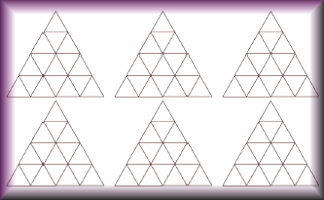Can you make up a puzzle like this for others to solve?

## Extension Challenge

How many triangles are on the front of this hotel reception desk?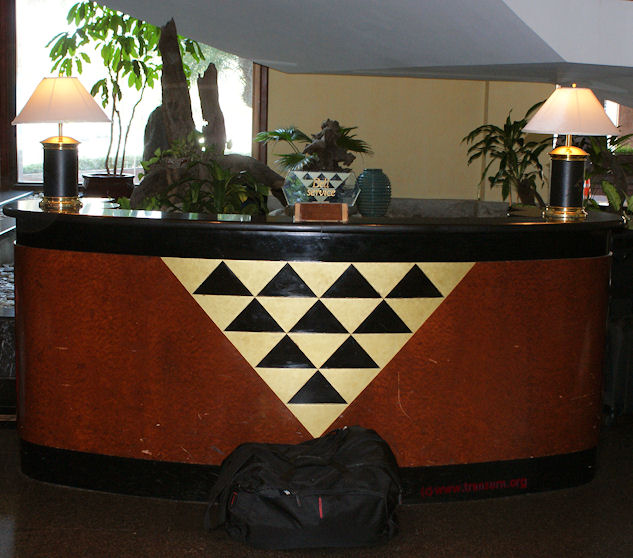Your access to the majority of the Transum resources continues to be free but you can help support the continued growth of the website by doing your Amazon shopping using the links on this page. Below is an Amazon search box and some items I have chosen and recommend to get you started. As an Amazon Associate I earn a small amount from qualifying purchases which helps pay for the upkeep of this website.

## GCSE Revision and Practice

Whatever exam board you use for GCSE Mathematics, this book by David Rayner remains an all-round winner. With this latest edition presented in full colour and completely updated for the new GCSE(9-1) specifications, this uniquely effective text continues to increase your chance of obtaining a good grade.

This book is targeted at the Higher tier GCSE, and provides a wealth of practice with careful progression, alongside substantial revision support for the new-style grading and exam questions. With all the new topics included, and a dedicated section on using and applying mathematics, this unique resource can be used either as a course book over two or three years or as a revision text in the run-up to exams. more...## Maths T-Shirts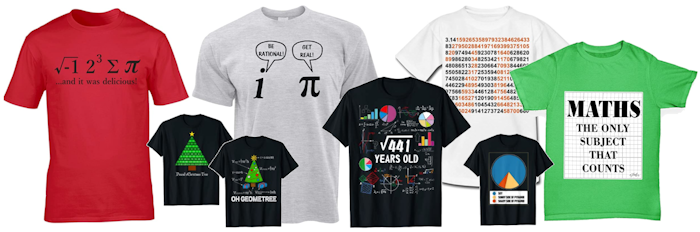Maths T-Shirts on AmazonOther shape counting starters:Teacher, do your students have access to computers?Do they have iPads or Laptops in Lessons? Whether your students each have a TabletPC, a Surface or a Mac, this activity lends itself to eLearning (Engaged Learning).Transum.org/go/?Start=September23

Here is the URL which will take them to a related student activity.

Transum.org/go/?to=howmany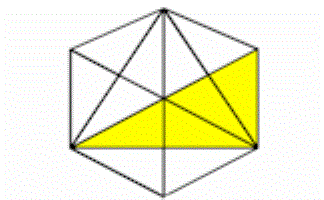For All: